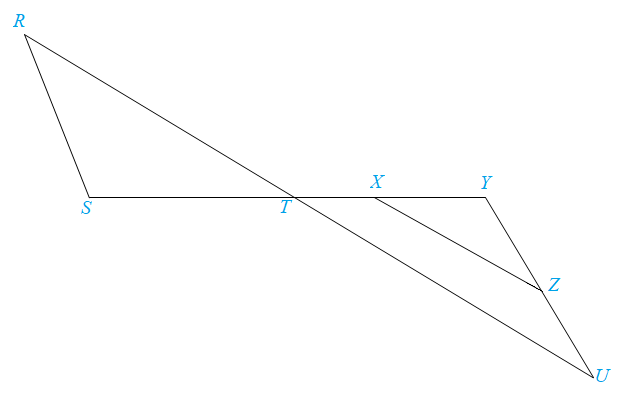Chapter 5.3, Problem 36EElementary Geometry For College St...

7th Edition
Alexander + 2 others
ISBN: 9781337614085

Solutions

Chapter
SectionElementary Geometry For College St...

7th Edition
Alexander + 2 others
ISBN: 9781337614085
Textbook Problem

Provide a paragraph proof for the following problem.Given: R S ¯ ∥ Y Z ¯ , R U ¯ ∥ X Z ¯ Prove: R S ⋅ Z X = Z Y ⋅ R TTo determine

To prove:

The statement RSZX=ZYRT if the sides RS and YZ are parallel, RS¯YZ¯ and the sides RU and XZ are parallel, RU¯XZ¯.

Explanation

Definition:

AA:

If two angles of one triangle are congruent to two angles of another triangle, then the triangles are similar.

CSSTP:

Corresponding sides of similar triangles are proportional.

Description:

Given that RS¯YZ¯,RU¯XZ¯.

The given figure is shown below.

Figure

From the given figure, it is observed that the sides RS and YZ are parallel, RS¯YZ¯.

If two parallel lines are cut by a transversal, then the alternate interior angles are congruent.

Therefore, RSTY.

From the given figure, it is also observed that the sides RU and XZ are parallel, RU¯XZ¯

Still sussing out bartleby?

Check out a sample textbook solution.

See a sample solution

The Solution to Your Study Problems

Bartleby provides explanations to thousands of textbook problems written by our experts, many with advanced degrees!

Get Started

What is the lowest score in the following distribution?

Essentials of Statistics for The Behavioral Sciences (MindTap Course List)

Solve the equations in Exercises 112 for x (mentally, if possible). x1=cx+d(c1)

Finite Mathematics and Applied Calculus (MindTap Course List)

Find the limit. lim0cos1sin

Single Variable Calculus: Early Transcendentals, Volume I

Prove, using Definition 6, that limx31(x+3)4= Definition 6

Single Variable Calculus: Early Transcendentals

True or False: f(x) = 10x − x2 is increasing on (4,8).

Study Guide for Stewart's Single Variable Calculus: Early Transcendentals, 8th

True or False: converges.

Study Guide for Stewart's Multivariable Calculus, 8th